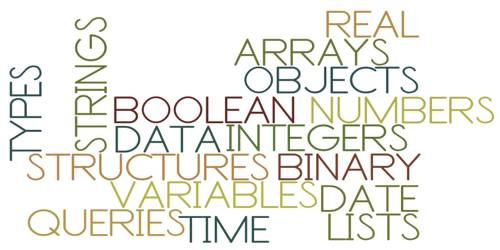# What are the four data types for sorting values in a program?

Data types: Programming uses a number of different data types. A data type determines what type of values and object can leave and what operation can be performed. A string, for example, is a data type that is used to classify text and an integer is a data type used to classify whole numbers.

(a) Character (String): One of the most widely used data types is the character. Here data are coded using character code such as EBCDIC or ASCII. Character or String consists of one or more character, which can include letters, numbers or other types of characters.

Example: [A, B, C, D, E, X, Y, Z]; [1, 2, 3, 4, 5, 6, 7, 8, 9, 0]; [<, >, +, -, *, /, \$, (, ), ?, !] etc.

(b) Real (Floating Point): Here numeric data are recorded using the exponential form of the data. Basically Real or Floating point data are fractional numbers. The data are converted into exponential form and then they are encoded in binary data.

Example: Given data, 0.092, Converted data: 9.2 EXP -2.

(c) Integer (Fixed Point): Here positive integers are coded using a binary representation and negative integers are coded by binary variation such as 2’s complement. An integer value is typically specified in the source code of a program as a sequence of digits optionally prefixed with + or −.

(d) Logical (Boolean Data): Logical data or Boolean data can represent two values: true or false. These two values are coded using one hit binary code;1 for true and 0 for false. The Boolean data type is mainly related to uncertain statements, which allow different actions by changing control flow depending on whether a programmer-specified Boolean situation evaluates to true or false.